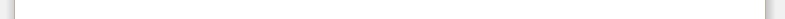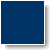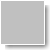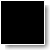Copyright © 2011 by "Mike Espig"   •   All Rights reserved   •   E-Mail: mike.espig@alopax.de
[Home] [Publications] [About] [Contact]
Homepage of Mike Espig
News:
 • Mai 2015: The approximation of tensors is important for the efficient numerical treatment of high dimensional problems, but it remains an extremely challenging task. One of the most popular approach to tensor approximation is the alternating least squares method. In our study, the convergence of the alternating least squares algorithm is considered. The analysis is done for arbitrary tensor format representations and based on the multiliearity of the tensor format. In tensor format representation techniques, tensors are approximated by multilinear combinations of objects lower dimensionality. The resulting reduction of dimensionality not only reduces the amount of required storage but also the computational effort. Our analysis is focused on global convergence and the rate of convergence. It is shown that the ALS method can converge sublinearly, Q-linearly, and even Q-superlinearly. Our theoretical results are illustrated on explicit examples, see On the Convergence of Alternating Least Squares Optimisation in Tensor Format Representations. • Jan. 2016: In this proof-of-principle study we apply tensor decomposition techniques to the Full Configuration Interaction (FCI) wavefunction in order to reduce the exponential scaling of the number of wavefunction parameters and overall computational effort. For this purpose, the wavefunction ansatz is formulated in an occupation number vector representation that ensures antisymmetry. If the canonical product format tensor decomposition is then applied, the Hamiltonian and the wavefunction can be cast into a multilinear product form. As a consequence, the number of wavefunction parameters does not scale to the power of the number of particles (or orbitals) but depends on the rank of the approximation and linearly on the number of particles. The degree of approximation can be controlled by a single threshold for the rank reduction procedure required in the algorithm. We demon- strate that using this approximation, the FCI Hamiltonian matrix and the wavefunction parameters can be stored with N^5 scaling and the FCI problem can be solved with subexponential effort. The error of the approximation that is introduced is below Millihartree for a threshold of e = 10-4 and no convergence problems are observed solving the FCI equations iteratively in the new format. While promising conceptually, all effort of the algorithm is shifted to the required rank reduction procedure after the contraction of the Hamiltonian with the coefficient tensor. At the current state, this crucial steps scales beyond N^10, see Tensor Representation Techniques for Full Configuration Interaction: A Fock Space Approach Using the Canonical Product Format.